ControlNet

## Self-Attention回顾

Transformer的核心是Self-Attention。Self-Attention是基于特征向量对序列上token成对关系的表征学习(Representation Learning)。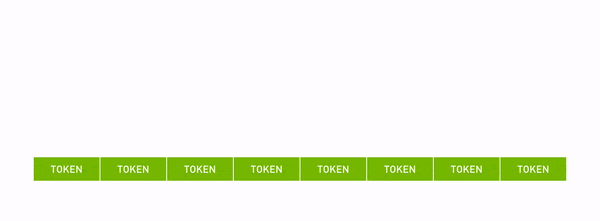Fig. 1. Self Attention.

• 序列: $X\in R^{n\times d}$
• Query向量: $Q$有相对应的可学习权重$W^Q\in R^{n\times dq}$
• Key向量: $K$也有相对应的可学习权重$W^K\in R^{n\times dk}$
• Value向量: $V$也有相对应的可学习权重$W^V\in R^{n\times dv}$
• $Q=XW^Q, K=XW^K, V=XW^V$
• Self-Attention层: $Z=softmax(\frac{QK^T}{\sqrt{d_q}})V$
• 若是Masked Self-Attention层，则需要增加一层mask: $softmax(\frac{QK^T}{\sqrt{d_q}}\circ M)$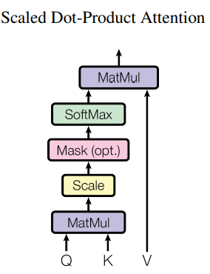Fig. 2. Self Attention运算过程. Adapted from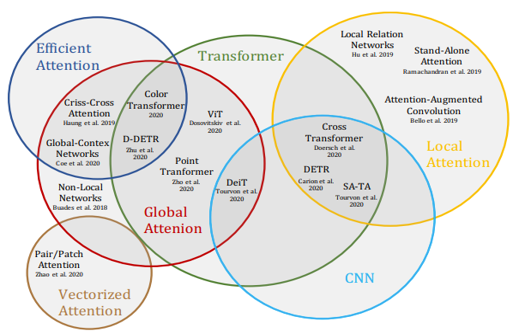Fig. 3. Self Attention不同类型. Adapted from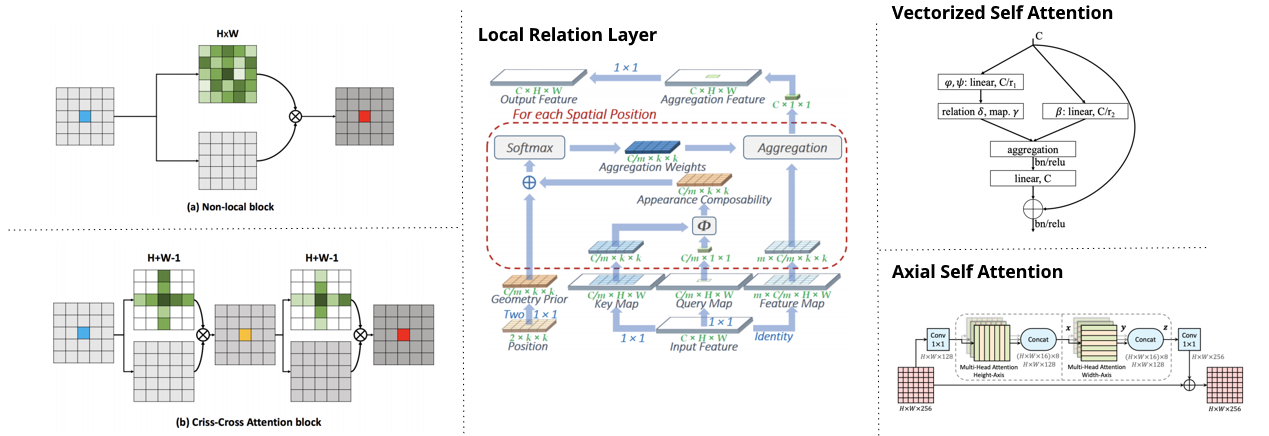Fig. 4. Self Attention的不同类型. Adapted from 

## 用Attention增强卷积

### Flatten和Attention层

• $H, W, F_in$是指特征图中高度、宽度、输入feature map的数量
• $N_h, d_v, d_k$是指head的数量，value和key的维度。其中$N_h$是可以整除$d_v$和$d_k$的。
• $d^h_v, d^h_k$是指每一个head中value和key的维度。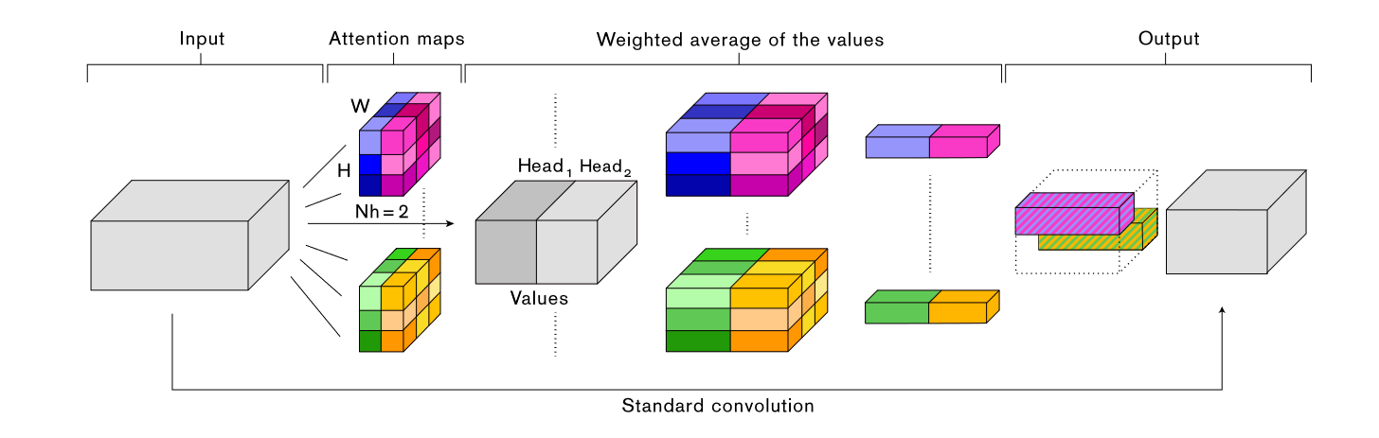Fig. 5. Attention Augmented Convolution的计算过程. Adapted from 

$$O_h=Softmax(\frac{(XW_q)(XW_k)^T}{\sqrt{d^h_k}})(XW_v)$$

### Positional Encoding

$$l_{i,j}=\frac{q_i^T}{\sqrt{d_k^h}}(k_j+r^W_{j_x-i_x}+r^H_{j_y-i_y})$$

$$O_h=Softmax(\frac{QK^T+S_H^{rel}+S_W^{rel}}{\sqrt{d^h_k}})V$$

$$AAConv(X)=Concat[Conv(X),MHA(X)]$$

### 实验结果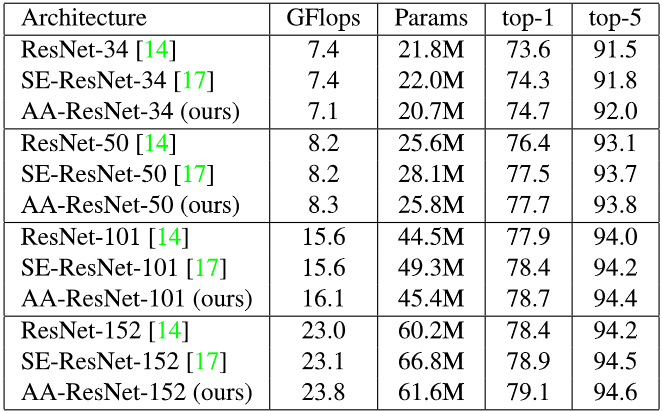Fig. 6. Attention Augmented用于分类的实验结果. Adapted from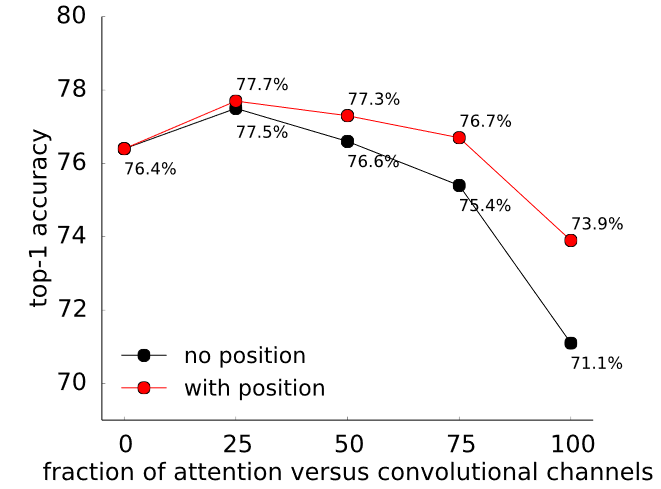Fig. 7. Positional Encoding是否使用的对比结果. Adapted from 

## E2E的Transformer对象检测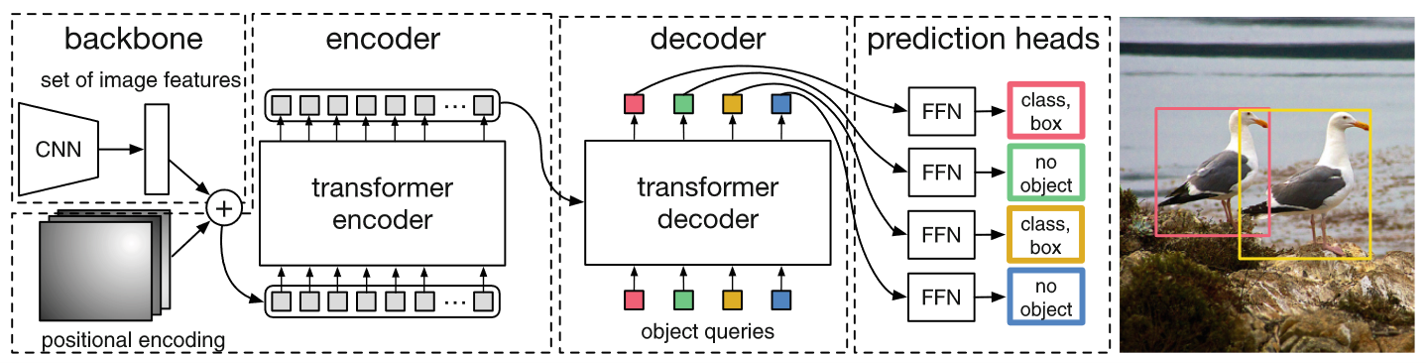Fig. 8. DETR结构. Adapted from 

### Transformer部分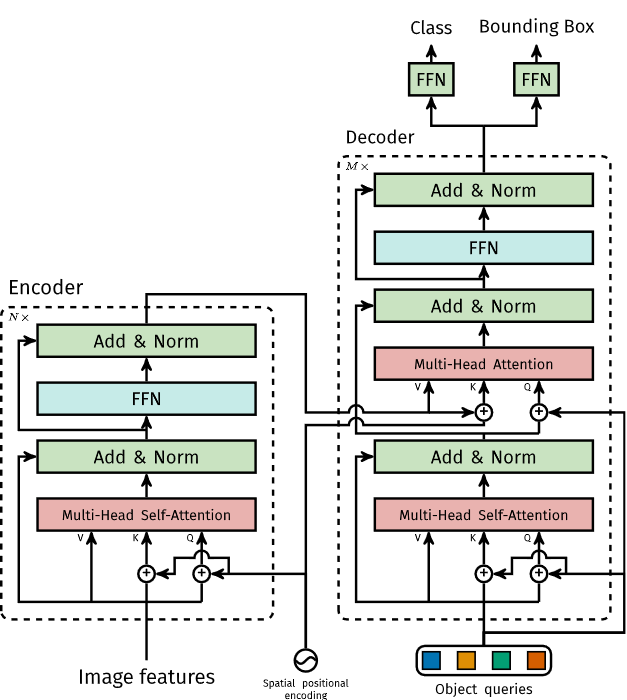Fig. 9. DETR中的Transformer部分. Adapted from 

### 损失函数

$$\hat{\sigma}=\mathop{\arg\min}_{\sigma\in\mathfrak{S}_N}\sum^N_i \mathcal{L}_{match}(y_i,\hat{y}_{\sigma(i)})$$

$$\mathcal{L}_{match}=-\mathbb{1}_{\{c_i\neq\emptyset\}}\hat{p}_{\sigma(i)}(C_i)+\mathbb{1}_{\{c_i\neq\emptyset\}}\mathcal{L}_{box}(b_i,\hat{b}_{\sigma(i)})$$

$$\mathcal{L}_{Hungarian}(y,\hat{y})=\sum^N_{i=1}[-log\hat{p}_{\hat{\sigma}(i)}(C_i)+\mathbb{1}_{\{c_i\neq\emptyset\}}\mathcal{L}_{box}(b_i,\hat{b}_{\hat{\sigma}}(i))]$$

$$\mathcal{L}=\lambda_{iou}\mathcal{L}_{iou}(b_i,\hat{b}_{\sigma(i)})+\lambda_{L1}||b_i-\hat{b}_{\sigma(i)}||_1$$

### 推断代码

Listing 1: DETR PyTorch推断代码. Adapted from 

### 实验结果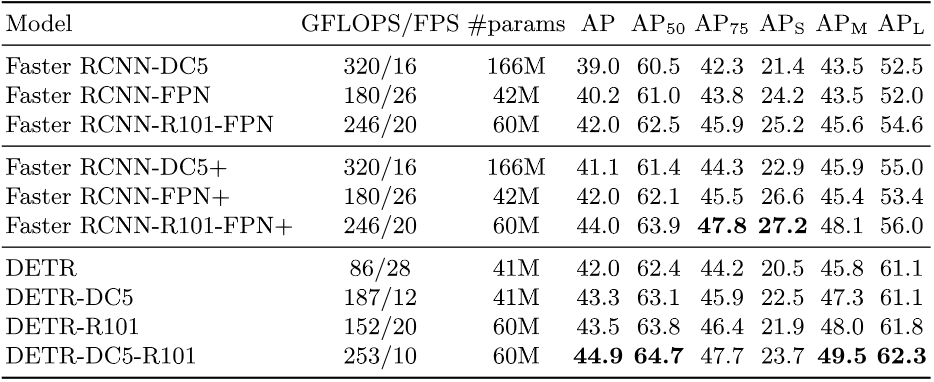Fig. 10. DETR实验结果. Adapted from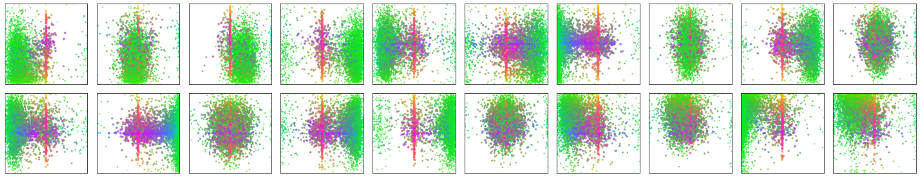Fig. 11. $N$个Object Queries的预测可视化. Adapted from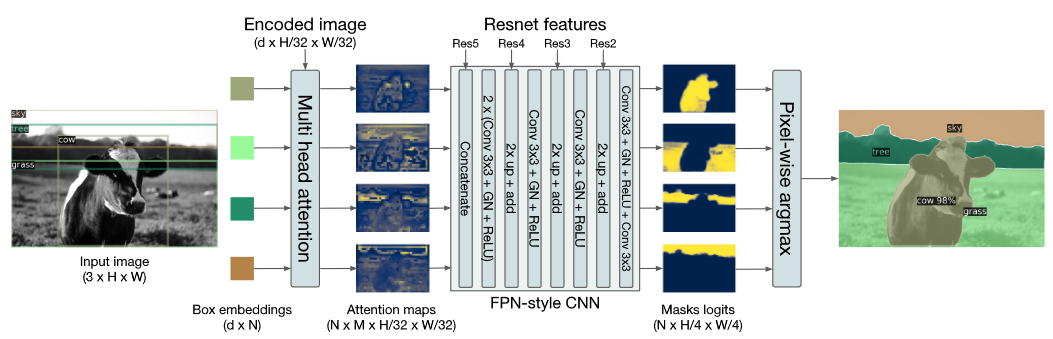Fig. 12. DETR用于图像分割. Adapted from 

## Transformer和知识蒸馏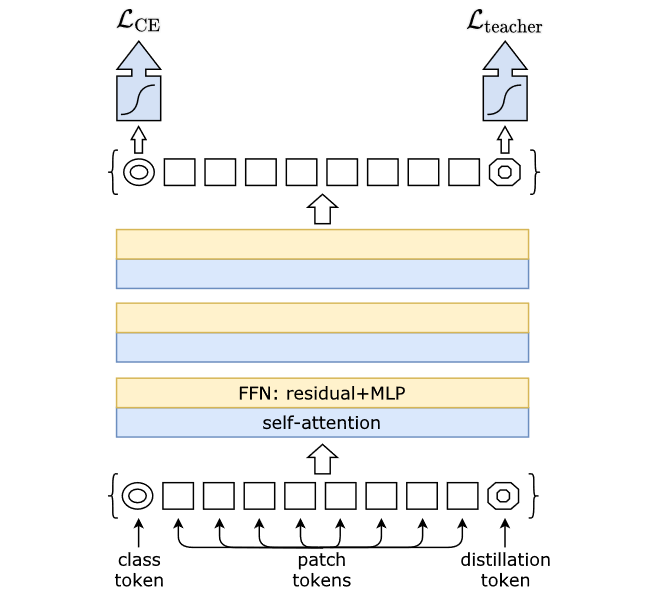Fig. 13. DeiT架构. Adapted from 

### 知识蒸馏

Fig. 13可以看出，相比于之前的ViT，它多了一个知识蒸馏的部分。首先是输入部分，除了左下角的class token之外，还增加了一个右下角的distillation token。这个distillation token经过Transformer会输出一个值用于计算蒸馏损失。

### 蒸馏损失

$$\mathcal{L}_{dis}^{hard}=\frac{1}{2}\mathcal{L}_{CE}(\psi(Z_s),y)+\frac{1}{2}\mathcal{L}_{CE}(\psi(Z_s),y_t)$$

$$\mathcal{L}_{dis}^{soft}=(1-\lambda)\mathcal{L}_{CE}(\psi(Z_s),y)+\lambda\tau^2KL(\psi(Z_s/\tau),\psi(Z_t/\tau))$$

### 教师模型的选择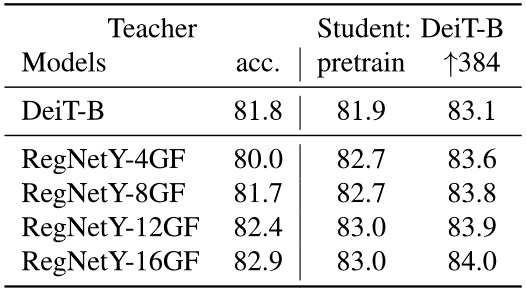Fig. 14. 教师模型的实验结果对比. Adapted from 

## 参考文献

•  A. Vaswani et al., “Attention is all you need,” in Proceedings of the 31st International Conference on Neural Information Processing Systems, Red Hook, NY, USA, Dec. 2017, pp. 6000–6010.
•  A. Dosovitskiy et al., “An Image is Worth 16x16 Words: Transformers for Image Recognition at Scale,” arXiv:2010.11929 [cs], Oct. 2020, [Online]. Available: http://arxiv.org/abs/2010.11929.
•  I. Bello, B. Zoph, A. Vaswani, J. Shlens, and Q. V. Le, “Attention Augmented Convolutional Networks,” in Proceedings of the IEEE/CVF International Conference on Computer Vision, 2019, pp. 3286–3295, [Online]. Available: https://openaccess.thecvf.com/content_ICCV_2019/html/Bello_Attention_Augmented_Convolutional_Networks_ICCV_2019_paper.html.
•  N. Carion, F. Massa, G. Synnaeve, N. Usunier, A. Kirillov, and S. Zagoruyko, “End-to-End Object Detection with Transformers,” in Computer Vision – ECCV 2020, Cham, 2020, pp. 213–229, doi: 10.1007/978-3-030-58452-8_13.
•  H. Touvron, M. Cord, M. Douze, F. Massa, A. Sablayrolles, and H. Jegou, “Training data-efficient image transformers & distillation through attention,” in International Conference on Machine Learning, Jul. 2021, pp. 10347–10357. Accessed: Jul. 22, 2021. [Online]. Available: http://proceedings.mlr.press/v139/touvron21a.html
•  S. Khan, M. Naseer, M. Hayat, S. W. Zamir, F. S. Khan, and M. Shah, “Transformers in Vision: A Survey,” arXiv:2101.01169 [cs], Feb. 2021, Accessed: Apr. 23, 2021. [Online]. Available: http://arxiv.org/abs/2101.01169
•  P. Shaw, J. Uszkoreit, and A. Vaswani, “Self-Attention with Relative Position Representations,” arXiv:1803.02155 [cs], Apr. 2018, Accessed: Jul. 25, 2021. [Online]. Available: http://arxiv.org/abs/1803.02155
•  H. Rezatofighi, N. Tsoi, J. Gwak, A. Sadeghian, I. Reid, and S. Savarese, “Generalized Intersection Over Union: A Metric and a Loss for Bounding Box Regression,” in Proceedings of the IEEE/CVF Conference on Computer Vision and Pattern Recognition, 2019, pp. 658–666. Accessed: Jul. 26, 2021. [Online]. Available: https://openaccess.thecvf.com/content_CVPR_2019/html/Rezatofighi_Generalized_Intersection_Over_Union_A_Metric_and_a_Loss_for_CVPR_2019_paper.html